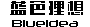您的位置：　首页 > 技术文档 > 多媒体制作 > Flash纯脚本生成饼图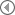APOLLO 未来畅想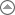回到列表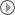基于flash的360虚拟现实引擎实现Flash纯脚本生成饼图
 作者：amu945　时间： 2007-03-17　文档类型：原创　来自：蓝色理想先发效果，效果有参考一下国外的饼图，不过他的代码，注释等我也看不懂，英文不懂，所以我就写个中文的好了。大家完全可以根据我的代码改出更多的效果来。编程水平有限，大家谅解一下，不过能正常运行。http://www.blueidea.com/articleimg/2007/03/4540/ok.swf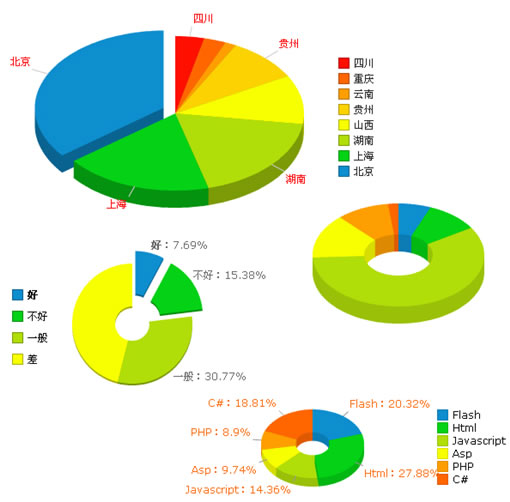数据来源于xml配置文件，可以直接修改xml文件以达到直接使用的目的。默认数据来源为piedata.xml但是可以在页面中修改这个数据来源。如下：代码拷贝框[Ctrl+A 全部选择 然后拷贝] 在代码中swf文件名后加上参数?piedata=piedata.asp则可以修改数据来源为piedata.asp。 其实原理也不难，先画个圆（扇形）做上表面，然后分别画出两个侧面和一个曲面，最后画底下的圆（扇形）。一个立体的小扇形就出来了。让他们一起拼在一起就成了饼图嘛。只有一点比较烦就是确定每一个扇形的深度，好让他们在视学上看起来处于正常的前后关系位置。在这儿，我想了一个法子。先算出当前扇形的中间度数，看这个度数是否在水平线以下，是的话就看这个度数与垂直方向的角度，角度最小的在最前面，最大的在最后面，如果中间度数在水平线以上，那么则与中间度数在水平线以下的相反。这儿要注意的是必须要从正上方作为0度，开始计算。下面是源文件，所有代码放到影片的时间轴上的一帧中就可以了。 代码拷贝框//缩放舞台并不缩放内容，将内容定位于舞台的左上角; Stage.align = "TL"; Stage.scaleMode = "noScale"; _quality = "BEST"; import mx.transitions.Tween; import mx.transitions.easing.*; //全局 //所有数据 var pie_num:String = "20,15,8,50,55,100,100,190"; //所有数据名称 var pie_name:String = "四川,重庆,云南,贵州,山西,湖南,上海,北京,广州"; //是否显示数据名称与百分比值 var show_name:String = "true,false,false,true,false,true,true,true" //所有颜色 var colorstr:String = "0xFF0F00,0xFF6600,0xFF9E01,0xFCD202,0xF8FF01,0xB0DE09,0x04D215,0x0D8ECF,0xFF1F11"; //所有开始位置 var defaultsate:String = "false,false,false,false,false,false,false,true"; //公共变量 x0 = 210;//圆点坐标x(数字) y0 = 135;//圆点坐标y(数字) z = 150;//圆长轴(>0) d = 90;//圆短轴(>0) w = 150;//环宽(>0,<长轴) h = 20;//饼高(>=0) movew = 15;//移动距离(>0) alpha = 100;//点击之后的透明度(0-100) mcalpha = 100;//默认所有开始透明度(0-100) showorder = true;//是否显示竖向排列表(true,false) orderx = 400;//竖向排列表起始x(数字) ordery = 70;//竖向排列表起始y(数字) orderxy = 6;//竖向排列表竖向间隔(>0) linecolor = 0xcccccc;//指示线颜色(十六进制颜色) linealpha = 100;//指示线透明度(0-100) titleline = 20; //指示线长度(>0 || 0<) fontcolor = 0xFF0000;//文字颜色(十六进制颜色) percentshow = "font";//是否显示百分值四个值（font文字,num百分值,all都显示,none不显） orderfontcolor = 0x222222;//左边竖向排列文字颜色(十六进制颜色) dark = 1.43;//深色系数（>1如1.43默认） //以下为三个饼图示例 //第一个参数为影片名，第二个为深度群 drawpie("amu",1, pie_num,pie_name,titleline,linecolor,linealpha,orderfontcolor,percentshow,fontcolor,colorstr,defaultsate,show_name, x0,y0,z,d,w,h,movew,alpha,mcalpha,dark, showorder,orderx,ordery,orderxy); drawpie("vapr",2, "15,30,60,90",",不好,一般,差,太差了",0,0xcccccc, 0,0x000000,"all",0x666666,"0x0D8ECF,0x04D215,0xB0DE09,0xF8FF01,0xFF9E01,0xFF6600,0xFF1F11","true,true,false,false","true,true,true,false", 160,380,70,70,50,2,15,100,100,1.5, true,20,340,13); drawpie("kiss",3, "30,50,290,70,50,10","Flash,Html,Javascript,Asp,PHP,C#",10,0x999999,100,0x000000,"none",0xff6600,"0x0D8ECF,0x04D215,0xB0DE09,0xF8FF01,0xFF9E01,0xFF6600,0xFF1F11","false,false,false,false,false,false,false","false,false,false,false,false,false,false", 470,300,100,60,60,20,15,50,100,1.15, false,280,250,28); drawpie("kisss",4, "242,332,171,116,106,224","Flash,Html,Javascript,Asp,PHP,C#",10,0x999999,100,0x000000,"all",0xff6600,"0x0D8ECF,0x04D215,0xB0DE09,0xF8FF01,0xFF9E01,0xFF6600,0xFF1F11","false,false,false,false,false,false,false","true,true,true,true,true,true,true", 370,520,60,40,40,10,15,50,100,1.15, true,515,480,3); function drawpie(mcname:String, depth:Number, pie_num:String, pie_name:String, titleline:Number, linecolor:Number,linealpha:Number, orderfontcolor:Number,percentshow:String, fontcolor:Number, colorstr:String, defaultsate:String, show_name:String, x0:Number, y0:Number, z:Number, d:Number, w:Number, h:Number, movew:Number, alpha:Number, mcalpha:Number, dark:Number, showorder:Boolean, orderx:Number, ordery:Number, orderxy:Number):Void{ arr_n = pie_num.split(","); arr_total=0; for(m=0;m180){ arr_middle[m] = 0.001; }else{ if((a-a0)/2+a0<=180 && (a-a0)/2+a0>=0){ //本mc的中间角度与90度的差值（这儿我们确定谁与90度差值的绝对值最小就排在最前面） arr_middle[m] = Math.abs(90-((a-a0)/2+a0)); }else if((a-a0)/2+a0>180 && (a-a0)/2+a0<=270){ arr_middle[m] = (Math.abs((a-a0)/2+a0-180)+500); //本mc的中间角度与180度的差值（这儿我们确定谁与90度差值的绝对值最大就排在最前面） }else if((a-a0)/2+a0>=-90 && (a-a0)/2+a0<0){ arr_middle[m] = (Math.abs((a-a0)/2+a0-360)+500); //本mc的中间角度与270度的差值（这儿我们确定谁与90度差值的绝对值最大就排在最前面） } } //trace(m+":"+arr_middle[m]); } //trace(arr_a); //扇环排序 arr_middle = arr_middle.sort(Array.NUMERIC|Array.RETURNINDEXEDARRAY);//重排序，让原来的数组值从小到大排列，但数组的索引不变。 //arr_middle.reverse(); // for(m = 0; m1如1.43) 本pie对应竖向排列方块mc名。 */ function drawCircle(mc:MovieClip, x0:Number, y0:Number, w1:Number, w2:Number, a0:Number, a:Number, h:Number, hw:Number, color:Number, movew:Number, alpha:Number, mcalpha:Number, movenow:String, valuepercent:Number, arr_name:String, arr_showname:String, titleline:Number, linecolor:Number,linealpha:Number, fontcolor:Number,percentshow:String,dark:Number, ordermc:MovieClip):Void { //加减间隔度 step = 1; //求内长短轴 leichang = w1-hw; leiduan = w2-(hw*w2/w1); //深色 hicolor = darkcolor(color,dark); //--------------------------------- //下面为下面环; mc.beginFill(color, 100); //mc.lineStyle(1, color, 100, false, "none"); //外边 mc.moveTo(getPoint(x0, y0+h, w1, w2, a0).x, getPoint(x0, y0+h, w1, w2, a0).y); k = a0; while (ka0) { k -= step; mc.lineTo(getPoint(x0, y0+h, leichang, leiduan, k).x, getPoint(x0, y0+h, leichang, leiduan, k).y); } //侧边2 mc.lineTo(getPoint(x0, y0+h, leichang, leiduan, a0).x, getPoint(x0, y0+h, leichang, leiduan, a0).y); mc.endFill(); //-------------------------------- //下边高 //下边外侧边高 if(a0<=0 && a>=0 && a<=180){ //解决90度时外侧面，显示事实为90度，实际为0度，因为我们整个旋转了-90度； mc.beginFill(hicolor, 100); mc.moveTo(getPoint(x0, y0+h, w1, w2, a0).x, getPoint(x0, y0+h, w1, w2, a0).y); k=a0; while (k<0) { k += step; mc.lineTo(getPoint(x0, y0+h, w1, w2, k).x, getPoint(x0, y0+h, w1, w2, k).y); } mc.lineTo(getPoint(x0, y0, w1, w2, 0).x, getPoint(x0, y0, w1, w2, 0).y); while (k>a0){ k -=step; mc.lineTo(getPoint(x0, y0, w1, w2, k).x, getPoint(x0, y0, w1, w2, k).y); } mc.endFill(); mc.beginFill(hicolor, 100); mc.moveTo(getPoint(x0, y0+h, w1, w2, 0).x, getPoint(x0, y0+h, w1, w2, 0).y); k=0; while (k0){ k -=step; mc.lineTo(getPoint(x0, y0, w1, w2, k).x, getPoint(x0, y0, w1, w2, k).y); } mc.endFill(); }else if(a>=180 && a0<=180 && a0>=0){ //解决180度时外侧面，显示事实为270度，实际为180度，因为我们整个旋转了-90度； mc.beginFill(hicolor, 100); mc.moveTo(getPoint(x0, y0+h, w1, w2, a0).x, getPoint(x0, y0+h, w1, w2, a0).y); k=a0; while (k<180) { k += step; mc.lineTo(getPoint(x0, y0+h, w1, w2, k).x, getPoint(x0, y0+h, w1, w2, k).y); } mc.lineTo(getPoint(x0, y0, w1, w2, 180).x, getPoint(x0, y0, w1, w2, 180).y); while (k>a0){ k -=step; mc.lineTo(getPoint(x0, y0, w1, w2, k).x, getPoint(x0, y0, w1, w2, k).y); } mc.endFill(); mc.beginFill(hicolor, 100); mc.moveTo(getPoint(x0, y0+h, w1, w2, 180).x, getPoint(x0, y0+h, w1, w2, 180).y); k=180; while (k180){ k -=step; mc.lineTo(getPoint(x0, y0, w1, w2, k).x, getPoint(x0, y0, w1, w2, k).y); } mc.endFill(); }else if(a0<=0 && a>=180){ //解决同时处于0度和180度的情况 mc.beginFill(hicolor, 100); mc.moveTo(getPoint(x0, y0+h, w1, w2, a0).x, getPoint(x0, y0+h, w1, w2, a0).y); k=a0; while (k<0) { k += step; mc.lineTo(getPoint(x0, y0+h, w1, w2, k).x, getPoint(x0, y0+h, w1, w2, k).y); } mc.lineTo(getPoint(x0, y0, w1, w2, 0).x, getPoint(x0, y0, w1, w2, 0).y); while (k>a0){ k -=step; mc.lineTo(getPoint(x0, y0, w1, w2, k).x, getPoint(x0, y0, w1, w2, k).y); } mc.endFill(); mc.beginFill(hicolor, 100); mc.moveTo(getPoint(x0, y0+h, w1, w2, 0).x, getPoint(x0, y0+h, w1, w2, 0).y); k=0; while (k<180) { k += step; mc.lineTo(getPoint(x0, y0+h, w1, w2, k).x, getPoint(x0, y0+h, w1, w2, k).y); } mc.lineTo(getPoint(x0, y0, w1, w2, 180).x, getPoint(x0, y0, w1, w2, 180).y); while (k>0){ k -=step; mc.lineTo(getPoint(x0, y0, w1, w2, k).x, getPoint(x0, y0, w1, w2, k).y); } mc.endFill(); mc.beginFill(hicolor, 100); mc.moveTo(getPoint(x0, y0+h, w1, w2, 180).x, getPoint(x0, y0+h, w1, w2, 180).y); k=180; while (k180){ k -=step; mc.lineTo(getPoint(x0, y0, w1, w2, k).x, getPoint(x0, y0, w1, w2, k).y); } mc.endFill(); }else{ mc.beginFill(hicolor, 100); mc.moveTo(getPoint(x0, y0+h, w1, w2, a0).x, getPoint(x0, y0+h, w1, w2, a0).y); k = a0; while (ka0) { k -= step; mc.lineTo(getPoint(x0, y0, w1, w2, k).x, getPoint(x0, y0, w1, w2, k).y); } mc.endFill(); } //下边开始侧面高 mc.beginFill(hicolor, 100); //mc.lineStyle(1, hicolor, 100, false, "none"); mc.moveTo(getPoint(x0, y0+h, w1, w2, a0).x, getPoint(x0, y0+h, w1, w2, a0).y); mc.lineTo(getPoint(x0, y0+h, leichang, leiduan, a0).x, getPoint(x0, y0+h, leichang, leiduan, a0).y); mc.lineTo(getPoint(x0, y0, leichang, leiduan, a0).x, getPoint(x0, y0, leichang, leiduan, a0).y); mc.lineTo(getPoint(x0, y0, w1, w2, a0).x, getPoint(x0, y0, w1, w2, a0).y); mc.endFill(); //下面结束侧面高 mc.beginFill(hicolor, 100); //mc.lineStyle(1, hicolor, 100, false, "none"); mc.moveTo(getPoint(x0, y0+h, w1, w2, a).x, getPoint(x0, y0+h, w1, w2, a).y); mc.lineTo(getPoint(x0, y0+h, leichang, leiduan, a).x, getPoint(x0, y0+h, leichang, leiduan, a).y); mc.lineTo(getPoint(x0, y0, leichang, leiduan, a).x, getPoint(x0, y0, leichang, leiduan, a).y); mc.lineTo(getPoint(x0, y0, w1, w2, a).x, getPoint(x0, y0, w1, w2, a).y); mc.endFill(); //下面内侧面高 mc.beginFill(hicolor, 100); //mc.lineStyle(1, hicolor, 100, false, "none"); mc.moveTo(getPoint(x0, y0+h, leichang, leiduan, a0).x, getPoint(x0, y0+h, leichang, leiduan, a0).y); k = a0; while (ka0) { k -= step; mc.lineTo(getPoint(x0, y0, leichang, leiduan, k).x, getPoint(x0, y0, leichang, leiduan, k).y); } mc.endFill(); //--------------------------------- //下面为上面环; mc.beginFill(color, 100); //mc.lineStyle(1, color, 100, false, "none"); //外边 mc.moveTo(getPoint(x0, y0, w1, w2, a0).x, getPoint(x0, y0, w1, w2, a0).y); k = a0; while (ka0) { k -= step; mc.lineTo(getPoint(x0, y0, leichang, leiduan, k).x, getPoint(x0, y0, leichang, leiduan, k).y); } //侧边2 mc.lineTo(getPoint(x0, y0, leichang, leiduan, a0).x, getPoint(x0, y0, leichang, leiduan, a0).y); mc.endFill(); //--------------------------------------显示名称与值 if(arr_showname=="true"){ mc.beginFill(color, 100); mc.lineStyle(1, linecolor, linealpha); mc.moveTo(getPoint(x0, y0, w1, w2, a0+(a-a0)/2).x,getPoint(x0, y0, w1, w2, a0+(a-a0)/2).y); mc.lineTo(getPoint(x0, y0, w1+titleline, w2+(w2*titleline)/w1, a0+(a-a0)/2).x,getPoint(x0, y0, w1+titleline, w2+(w2*titleline)/w1, a0+(a-a0)/2).y); mc.endFill(); //trace(mc._name+":"+(a0+(a-a0)/2)); if(a0+(a-a0)/2>0 && a0+(a-a0)/2<90){ var label:TextField = mc.createTextField("label", 1, getPoint(x0, y0, w1+titleline, w2+(w2*titleline)/w1, a0+(a-a0)/2).x, getPoint(x0, y0, w1+titleline, w2+(w2*titleline)/w1, a0+(a-a0)/2).y, 0, 0); label.autoSize = "left"; }else if(a0+(a-a0)/2>90 && a0+(a-a0)/2<180){ var label:TextField = mc.createTextField("label", 1, getPoint(x0, y0, w1+titleline, w2+(w2*titleline)/w1, a0+(a-a0)/2).x, getPoint(x0, y0, w1+titleline, w2+(w2*titleline)/w1, a0+(a-a0)/2).y, 0, 0); label.autoSize = "right"; }else if(a0+(a-a0)/2>180 && a0+(a-a0)/2<270){ var label:TextField = mc.createTextField("label", 1, getPoint(x0, y0, w1+titleline, w2+(w2*titleline)/w1, a0+(a-a0)/2).x, getPoint(x0, y0, w1+titleline, w2+(w2*titleline)/w1, a0+(a-a0)/2).y-18, 0, 0); label.autoSize = "right"; }else if(a0+(a-a0)/2>-90 && a0+(a-a0)/2<0){ var label:TextField = mc.createTextField("label", 1, getPoint(x0, y0, w1+titleline, w2+(w2*titleline)/w1, a0+(a-a0)/2).x, getPoint(x0, y0, w1+titleline, w2+(w2*titleline)/w1, a0+(a-a0)/2).y-18, 0, 0); label.autoSize = "left"; }else if(a0+(a-a0)/2==0){ var label:TextField = mc.createTextField("label", 1, getPoint(x0, y0, w1+titleline, w2+(w2*titleline)/w1, a0+(a-a0)/2).x, getPoint(x0, y0, w1+titleline, w2+(w2*titleline)/w1, a0+(a-a0)/2).y-9, 0, 0); label.autoSize = "left"; }else if(a0+(a-a0)/2==90){ var label:TextField = mc.createTextField("label", 1, getPoint(x0, y0, w1+titleline, w2+(w2*titleline)/w1, a0+(a-a0)/2).x, getPoint(x0, y0, w1+titleline, w2+(w2*titleline)/w1, a0+(a-a0)/2).y, 0, 0); label.autoSize = "center"; }else if(a0+(a-a0)/2==180){ var label:TextField = mc.createTextField("label", 1, getPoint(x0, y0, w1+titleline, w2+(w2*titleline)/w1, a0+(a-a0)/2).x, getPoint(x0, y0, w1+titleline, w2+(w2*titleline)/w1, a0+(a-a0)/2).y-9, 0, 0); label.autoSize = "right"; } label.html = true; if(percentshow=="all"){ label.htmlText = arr_name+"："+valuepercent+"%"; }else if(percentshow=="font"){ label.htmlText = arr_name; }else if(percentshow=="num"){ label.htmlText = valuepercent+"%"; } var myformat:TextFormat = new TextFormat(); myformat.font = "Verdana"; myformat.size = 12; myformat.color = fontcolor; label.setTextFormat(myformat); label.gridFitType = "pixel"; label.antiAliasType = "advanced"; label.sharpness = -400; } //-------------------------------------- //点击移动 mc.onRelease = function(){ //移动方向：度数： go_a = a0+(a-a0)/2; //移动距离 go_w = movew; //trace(this._name); //trace(this.enabled); if(mc._x!=0 || mc._y!=0){ myTweeen3=new Tween(mc,"_x",Bounce.easeOut,mc._x,(mc._x - (getPoint(x0,y0,go_w,go_w,go_a).x-x0)),1.5,true); myTweeen4=new Tween(mc,"_y",Bounce.easeOut,mc._y,(mc._x - (getPoint(x0,y0,go_w,go_w,go_a).x-x0)),1.5,true); //透明度 myTweeenalpha2=new Tween(mc,"_alpha",Regular.easeOut,mc._alpha,mcalpha,1,true); var listener3:Object = new Object(); mc.enabled = false; ordermc.enabled = false;//竖向排列本pie对应的方块mc listener3.onMotionFinished = function(){ mc.enabled = true; ordermc.enabled = true;//竖向排列本pie对应的方块mc } myTweeen3.addListener(listener3); //mc._x = mc._x - (getPoint(x0,y0,go_w,go_w,go_a).x-x0); //mc._y = mc._y - (getPoint(x0,y0,go_w,go_w,go_a).y-y0); }else{ //到达新点 myTweeen1=new Tween(mc,"_x",Regular.easeOut,mc._x,(getPoint(x0,y0,go_w,go_w,go_a).x-x0),1,true); myTweeen2=new Tween(mc,"_y",Regular.easeOut,mc._y,(getPoint(x0,y0,go_w,go_w,go_a).y-y0),1,true); //透明度 myTweeenalpha=new Tween(mc,"_alpha",Regular.easeOut,mc._alpha,alpha,1,true); var listener1:Object = new Object(); mc.enabled = false; ordermc.enabled = false;//竖向排列本pie对应的方块mc listener1.onMotionFinished = function(){ mc.enabled = true; ordermc.enabled = true;//竖向排列本pie对应的方块mc } myTweeen1.addListener(listener1); } //trace(this.getDepth()); } //默认位置（移动） if(movenow=="true"){ //移动方向：度数： go_a = a0+(a-a0)/2; //移动距离 go_w = movew; myTweeen5=new Tween(mc,"_x",Regular.easeOut,mc._x,(getPoint(x0,y0,go_w,go_w,go_a).x-x0),1,true); myTweeen6=new Tween(mc,"_y",Regular.easeOut,mc._y,(getPoint(x0,y0,go_w,go_w,go_a).y-y0),1,true); //透明度 myTweeenalpha=new Tween(mc,"_alpha",Regular.easeOut,mc._alpha,alpha,1,true); var listener5:Object = new Object(); mc.enabled = false; ordermc.enabled = false;//竖向排列本pie对应的方块mc listener5.onMotionFinished = function(){ mc.enabled = true; ordermc.enabled = true;//竖向排列本pie对应的方块mc } myTweeen5.addListener(listener5); } } /*-------------------------------------------------------------------------------------------- x=a*cosX //x、y是椭圆上一点的坐标；a、b分别是长、短轴 y=b*sinX //X是椭圆旋转的角度。 计算椭圆上点的位置函数，x0，y0为圆心；w，h为长短轴；a为度数。返回一个坐标对象，有x和y两个属性。 */ function getPoint(x0:Number, y0:Number, w:Number, h:Number, a:Number):Object { a = a*Math.PI/180; return {x:Math.cos(a)*w+x0, y:Math.sin(a)*h+y0}; } //-------------------------------------------------------------------------------------- /*-------------------------------------------------------------------------------------------- 将颜色加深 原有颜色oldcolor为十六进表示法如：0xff6600;返回同样类型的值 */ function darkcolor(oldcolor:Number,dark:Number):Number{ //求暗色 if(oldcolor.toString(16).length<6){//防止这个十六进制数的第一位为0; r = parseInt("0x0"+substring(oldcolor.toString(16), 0, 1)); g = parseInt("0x"+substring(oldcolor.toString(16), 2, 2)); b = parseInt("0x"+substring(oldcolor.toString(16), 4, 2)); }else{ r = parseInt("0x"+substring(oldcolor.toString(16), 0, 2)); g = parseInt("0x"+substring(oldcolor.toString(16), 3, 2)); b = parseInt("0x"+substring(oldcolor.toString(16), 5, 2)); } //trace("原始rgb:"+r+":"+g+":"+b); //dark = 1.43; r=Math.round(r/dark).toString(16); g=Math.round(g/dark).toString(16); b=Math.round(b/dark).toString(16); //trace("新rgb:"+r+":"+g+":"+b); r.length==1 ? r="0"+r:r; g.length==1 ? g="0"+g:g; b.length==1 ? b="0"+b:b; var hicolor:Number; hicolor = parseInt("0x"+r.toString(16)+""+g.toString(16)+""+b.toString(16)); r = parseInt("0x"+substring(hicolor.toString(16), 0, 2)); g = parseInt("0x"+substring(hicolor.toString(16), 3, 2)); b = parseInt("0x"+substring(hicolor.toString(16), 5, 2)); return hicolor; }[Ctrl+A 全部选择 然后拷贝] 源文件打包下载 flashpie.rar 本文链接：http://www.blueidea.com/tech/multimedia/2007/4540.asp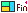出处：蓝色理想 责任编辑：feng4ever ◎进入论坛RIA设计与应用版块参加讨论相关文章 更多相关链接通过实例学习AS3.0――案例六Tracer-Flash代码调试类通过实例学习AS3.0――案例五通过实例学习AS3.0――案例四通过实例学习AS3.0――案例三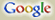全网 本站 论坛
 热门搜索：CSS Fireworks 设计比赛 网页制作 web标准 用户体验 UE photoshop Dreamweaver Studio8 Flash 手绘 CG站点最新 站点最新列表周大福“敬•自然”设计大赛开启国际体验设计大会7月将在京举行中国国防科技信息中心标志征集云计算如何让安全问题可控云计算是多数企业唯一拥抱互联网的机会阿里行云云手机年终巨献，送礼标配299起阿里巴巴CTO王坚的"云和互联网观"1499元买真八核 云OS双蛋大促首届COCO桌面手机主题设计大赛栏目最新 栏目最新列表浅谈JavaScript编程语言的编码规范如何在illustrator中绘制台历Ps简单绘制一个可爱的铅笔图标数据同步算法研究用ps作简单的作品展示页面CSS定位机制之一:普通流25个最佳最闪亮的Eclipse开发项目Illustrator中制作针线缝制文字效果Photoshop制作印刷凹凸字体VS2010中创建自定义SQL Rule分享按钮 蓝色理想版权申明：除部分特别声明不要转载，或者授权我站独家播发的文章外，大家可以自由转载我站点的原创文章，但原作者和来自我站的链接必须保留（非我站原创的，按照原来自一节，自行链接）。文章版权归我站和作者共有。 转载要求：转载之图片、文件，链接请不要盗链到本站，且不准打上各自站点的水印，亦不能抹去我站点水印。 特别注意：本站所提供的摄影照片，插画，设计作品，如需使用，请与原作者联系，版权归原作者所有，文章若有侵犯作者版权，请与我们联系，我们将立即删除修改。・不良评论请用报告管理员，以利管理员及时删除。
・尊重网上道德，遵守中华人民共和国的各项有关法律法规
・承担一切因您的行为而直接或间接导致的民事或刑事法律责任
・本站评论管理人员有权保留或删除其管辖评论中的任意内容
・您在本站发表的作品，本站有权在网站内转载或引用
・参与本评论即表明您已经阅读并接受上述条款专业书推荐 更多内容网站可用性测试及优化指南《写给大家看的色彩书1》《跟我去香港》众妙之门―网站UI 设计之道《Flex 4.0 RIA开发宝典》《赢在设计》犀利开发―jQuery内核详解与实践作品集 更多内容